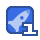# Java斗牛和投筛子【终极版】8收藏

System.out.println("已进入游戏 ， 按1继续 退出请按 2 "); int t = scanner.nextInt(); if (t == 2) { System.out.println("已退出 "); break; } int money = 1000; int yuanbao = 10; double a = 0, b = 0, c = 0, d = 0, e = 0, f = 0; while (true) { int max = 6; int min = 1; int number = (int) (Math.random() * (max + 1 - min) + min); System.out .println("请选择 开始游戏

...展开详情

• 8
资源
• 2
粉丝
•等级
•分享达人Java斗牛和投筛子【终极版】 5积分/C币 立即下载
1/0